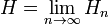Spring 2012

# Jan 26

## from last class

For sequence of characters with independent probabilities, e.g.
 a b a c a b a c ... , generated only by P(a), P(b), P(c) H = - sum p(x) * log(p(x)) 
Shannon, pg 10 :
 This works for a single random choice as well: choice F = (p1=1/2, p2=1/3, p3=1/6). Calculate then entropy H(1/2, 1/3, 1/6). This is the *same* as the entropy of that one choice "broken into two steps", i.e. first a (1/2, 1/2) choice, then a (2/3, 1/3) choice 50% of the time, which is H(1/2, 1/2) + 0.5 H(2/3, 1/3). Show explicitly that our entropy formula gives H(1/2, 1/3, 1/6) = H(1/2, 1/2) + 0.5 H(2/3, 1/3) ... which is in part how Shannon motivates the formula for H. 

## new stuff

Suppose we have two random choices F, G :
• What is (or should be, or definition of) the entropy H(F & G)?
• How does that compare to the individual entropies, H(F), H(G)?
• How should we define things if one depends on the other
 P(G|F) = P(F & G)/P(F) 
If we have a markov order 1 chain of symbols (using that conditional probability notion),with probability of each symbol depending on the one previous, how *should* we define the "entropy per symbol" so that it has the same intuition as we had with the markov 0 chain?
See how much can be "guessed" from looking at some dirt simple examples.
Stop here, work some examples, and think hard ...

Result that we want to get :
 H = entropy treating source as markov-0 = - sum p(x) log[p(x)] H = entropy treating source as markov-1 = -sum p(xy) log[p(y|x)] , sum over all "xy" pairs = H[y|x] i.e. conditional entropy H = entropy treating source as markov-2 = -sum p(xyz) log[p(z|xy)], sum over all "xyz" triples 
and that the "true" entropy (and compressibility) of an arbitrary source iswith definitions
 H(y | x) = − ∑ p(x,y)log(p(y | x)) x,y
Hn = H(y | x1x2...xn) for markov-n model
 p(x,y) is joint probability of of x & y, i.e. p("xy") 2-tuple p(y|x) is conditional probability of y given a specific x. 
See my my entropy notes and code examples. (Note: there may well be errors in either - confirm as you read!)
Your mission: write some code to actually implement this calculation, to ensure you understand exactly what the formula means.
http://cs.marlboro.edu/ courses/ spring2012/information/ notes/ Jan_26